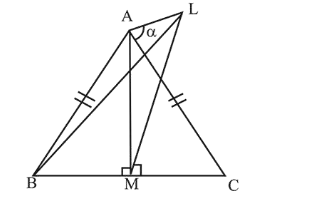# ABC is a triangular park with

Question:

$\mathrm{ABC}$ is a triangular park with $\mathrm{AB}=\mathrm{AC}=100$ metres. $\mathrm{A}$ vertical tower is situated at the mid-point of $\mathrm{BC}$. If the angles of elevation of the top of the tower at $A$ and $B$ are $\cot ^{-1}(3 \sqrt{2})$ and $\operatorname{cosec}^{-1}(2 \sqrt{2})$ respectively, then the height of the tower (in metres) is :

1. (1) $\frac{100}{3 \sqrt{3}}$

2. (2) $10 \sqrt{5}$

3. (3) 20

4. (4) 25

Correct Option: , 3

Solution:

Let the height of the vertical tower situated at the mid point of BC be $h$.In $\triangle \mathrm{ALM}$,

$\cot \mathrm{A}=\frac{A M}{L M}$

$\Rightarrow 3 \sqrt{2}=\frac{A M}{h} \Rightarrow \mathrm{AM}=3 \sqrt{2} h$

In $\triangle \mathrm{BLM}$,

$\cot \mathrm{B}=\frac{B M}{L M} \Rightarrow \sqrt{7}=\frac{B M}{h} \Rightarrow \mathrm{BM}=\sqrt{7} h$

In $\triangle \mathrm{ABM}$ by Pythagoras theorem

$\mathrm{AM}^{2}+\mathrm{MB}^{2}=\mathrm{AB}^{2}$

$\therefore \mathrm{AM}^{2}+\mathrm{MB}^{2}=(100)^{2}$

$\Rightarrow 18 h^{2}+7 h^{2}=100 \times 100$

$\Rightarrow h^{2}=4 \times 100 \Rightarrow h=20$